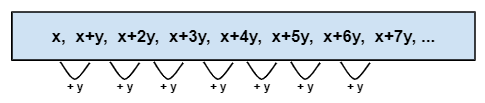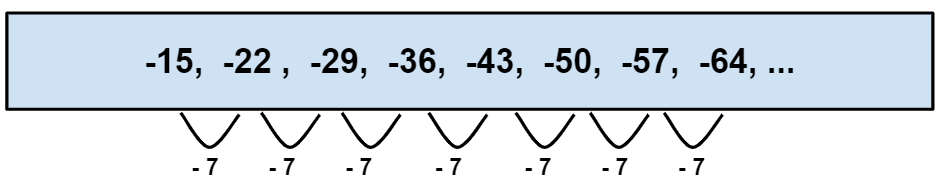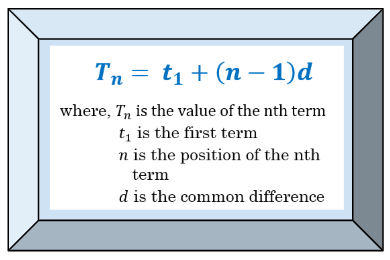# Arithmetic Sequence

TOPIC: ARITHMETIC SEQUENCE/ARITHMETIC PROGRESSION

Sub-topics:

• Arithmetic Means
• Word Problems Involving Arithmetic Sequence

LEARNING OBJECTIVES:

• To recognise arithmetic sequence
• To illustrate arithmetic means
• To solve problems involving arithmetic sequence

MATH CONCEPTS

• ARITHMETIC SEQUENCE – It is a sequence in which the difference between any two consecutive terms is equal.
• COMMON DIFFERENCE – It is the constant difference of any two consecutive terms (nth term minus the previous term) in an arithmetic sequence. Thus,$d = t_{2}-t_{1}=t_{3}-t_{2}=t_{n}-t_{n-1}$
• FINDING THE NTH TERM OF AN ARITHMETIC SEQUENCE:$T_{n} = t_{1} + (n-1)d$ where$T_{n}$ is the value of the nth term,$t_{1}$ is the first term, n is the position of the nth term, and d is the common difference.
• ARITHMETIC MEANS – It is the average of two terms in an arithmetic sequence.

IMPORTANCE

• Arithmetic sequence is a functional notation. Each element in the domain has a unique corresponding element in the range.
• Understanding the concepts of arithmetic sequence is already a good prerequisite knowledge in understanding linear functions.

DISCUSSION

• ARITHMETIC SEQUENCE

Arithmetic sequence is a special type of sequence where there is a constant difference present in any two consecutive terms. This common difference can be any real number.In the given example, notice that each term is being added constantly by y. The answer would still be the same if you solve for the difference of any two consecutive terms. In this sequence, the first term,$t_{1} = x$, the common difference d = y, and the number of given terms n = 8.Remember, the common difference can also be a negative number. Here, the arithmetic sequence is decreasing and the common difference is equal to -7.  In this sequence, the first term,$t_{1} = -15$, the common difference d = -7, and the number of given terms n = 8.

ILLUSTRATIVE EXAMPLES

Identify whether the following sequences are arithmetic sequences. If it is, solve for the common difference.

1) 1, 2, 3, 4, 6

2) π, 2π+3, 3π+6, 4π+9

3) 1/8, 1/4, 3/8, 1/2

SOLUTIONS:

1) To say that a sequence is an example of arithmetic sequence, any two consecutive terms must have a common difference. Notice that from$t_{2}-t_{1},\, t_{3}-t_{2},\,t_{4}-t_{3}$ is equal to 1 but$t_{5}-t_{4} = 2$. In that case, 1, 2, 3, 4, 6 is not an arithmetic sequence.

2) Investigate if there is a constant number between two consecutive terms.Since there is a constant difference ( π + 3 ), this is an arithmetic sequence.

3) Investigate if there is a constant number between two consecutive terms.Since there is a constant difference ( d = 18 ), this is an arithmetic sequence.

DISCUSSION

• FINDING THE NTH TERM OF AN ARITHMETIC SEQUENCE AND ARITHMETIC MEANS

To find the nth term of an arithmetic sequence, use the formulaIt is very important to identify the given values in order to arrive with the correct answer. The formula is very easy to use and to follow.

ILLUSTRATIVE EXAMPLES

Solve for the following arithmetic sequence-related problems.

1) Find the 16th term of an arithmetic sequence if the first term is 8 and d= – 9.

Using the formula of finding the nth term of an arithmetic sequence:$T_{n} = t_{1}+(n-1)d$$T_{16} = ?$$T_{1} = 8$$d=9$$T_{16} = 8+[(16-1) (-9)]$$T_{16} = 8+[(15) (-9)]$$T_{16} = 8 - 135$$T_{16} = -127$

2) Find the value of x if the first term is x, d = 3x, and the 9th term is 25.

Using the formula of finding the nth term of an arithmetic sequence:$T_{9} = 25$$T_{1} = x$$d = 3x$$T_{9} = x+[(9-1) (3x)]$

25 = x + (8)(3x)

25 = x + (24x)

25 = 25x

1 = x

3) Find the missing terms of the arithmetic sequence ___, 6, ____, ____, 30.

To solve this problem, we need our knowledge about arithmetic means.  We should solve for the common difference to complete the terms of the sequence. Using the formula of finding the nth term of an arithmetic sequence:$T_{n} = t_{1}+(n-1)d$, let’s derive the formula for the common difference.$T_{n} = t_{1}+(n-1)d$$T_{n}-t_{1} =(n-1)d$

(Addition Property of Equality: add$-t_{1}$ to both sides of the equation)$\frac{T_{n}-t_{1}}{(n-1)}=d$

(Multiplication Property of Equality: Multiply both sides by the reciprocal of n-1)$\frac{T_{n}-t_{1}}{(n-1)}=d$

(The formula for common difference)

In this arithmetic sequence ___, 6, ____, ____, 30, Let 6 be the first term and 30 will now be the 4th term. Using the derived formula for d,$\frac{T_{n}-t_{1}}{(n-1)}=d$$\frac{30-6}{(4-1)}=d$$\frac{24}{3}=d$

8 = d

Since we already know the value for d, we can now supply the missing terms in the sequence. The complete sequence is -2, 6, 14, 22, 30 .

4) An employer offers an expert a beginning salary of £ 40, 300 per year. If the salary is increased to £ 1200 yearly, what would be the expert’s salary in his 5th year of working?

Using the formula of finding the nth term of an arithmetic sequence:$T_{n}=t_{1}+(n-1)d$.$T_{5} = ?$$T_{1} = 40, 300$$d= 1,200$$T_{n}=t_{1}+(n-1)d$$T_{5}= 40,300+(5-1) 1200$$T_{5}= 40,300+(4) 1200$$T_{5}= 40,300+4,800$$T_{5}= 45,100$

Therefore, his 5th year salary will be £ 45, 100.

REFERENCES:

https://www.mathsisfun.com/algebra/sequences-sums-arithmetic.html